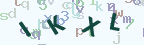### Java 中如何用 Math.random() 生成一个随机数

##### 本文通过实例演示1到99之间的随机数，100到999之间的随机数，100000到999999之间的随机数是如何生成的。

random() 返回一个带正号的 double 类型的值，该值 大于等于 0.0，而且小于 1.0。

```// Math.random()随机数的值
double d01 = Math.random();
System.out.println("Math.random()随机数的值：" + d01);

// 输出 1 到 99 之间的随机数，思路如下：
// [1, 99] ---> [0, 98] +　1
// Math.random() ---> [0.0, 1.0)
// Math.random()*99 ---> [0.0, 99.0)
// (int)(Math.random()*99) ---> [0, 98]
// (int)(Math.random()*99)+1 ---> [1, 99]
int i07 = (int)(Math.random()*99) + 1;
System.out.println("输出 1 到 99 之间的随机数：" + i07);

// 输出 100 到 999 之间的随机数，思路如下：
// [100, 999] ---> [0, 899] + 100
// Math.random() ---> [0.0, 1.0)
// Math.random()*999 ---> [0.0, 999.0)
// (int)(Math.random()*999) ---> [0, 998]
// (int)(Math.random()*900)+100 ---> [100, 999]
int i08 = (int)(Math.random()*900) + 100;
System.out.println("输出 100 到 999 之间的随机数：" + i08);

// 输出 100000 到 999999 之间的随机数，思路如下：
// [100000, 999999] ---> [0, 899999] + 100000
// Math.random() ---> [0.0, 1.0)
// Math.random()*999999 ---> [0.0, 999999.0)
// (int)(Math.random()*999999) ---> [0, 999998]
// (int)(Math.random()*899999)+100000 ---> [100000, 999999]
int i09 = (int)(Math.random()*899999) + 100000;
System.out.println("输出 100000 到 999999 之间的随机数：" + i09);```#### 评论列表

 暂时没有相关记录

#### 发表评论

换一张？